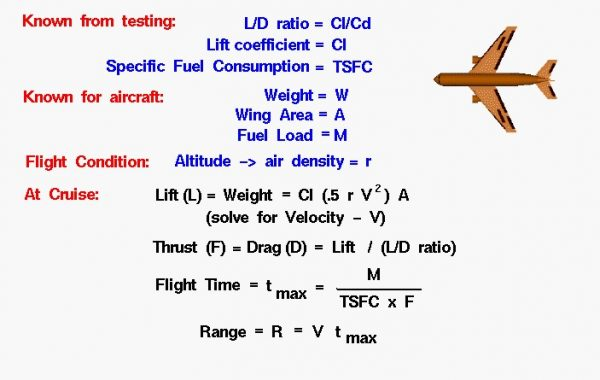# Range Summary## Cruise Conditions of an Aircraft’s Range

Let’s summarize the information necessary to do a preliminary calculation of an aircraft’s range under cruise conditions. We are taking a very simple view of aircraft range – for academic purposes. In reality, calculating the range is a complex problem because of the large number of variables. An aircraft’s flight is not conducted at a single ground speed but varies from zero at take-off, to cruise conditions, and back to zero at landing. Extra fuel is expended in climbing to altitude and in maneuvering the aircraft. The weight is constantly changing as fuel is burned, so the lift, drag, and thrust and fuel consumption rate are also continually changing. On real aircraft, just like with your automobile, there is usually a fuel reserve, and the pilot makes sure to land the plane with fuel still on board. We are going to neglect all of these effects.

## Wind Tunnel Testing of an Aircraft

There are certain things that we need to know about our aircraft. From previous wind tunnel testing, we need to determine the L/D ratio Cl/Cd and the lift coefficient Cl. We also need some information about the propulsion system, specifically, the specific fuel consumption rate TSFC of the engine. For the aircraft itself, we need to know the weight W of the aircraft, the wing area, A and the fuel load M. We are free to select a flight altitude, but this determines the air density r from our model of the standard atmosphere.

## Lift Equation

In cruise, the lift L is equal to the weight W and the thrust F is equal to the drag D. Using the lift equation, we can solve for the velocity necessary to create enough lift to equal the weight.

## Velocity

In this equation, all of the variables are known except the velocity V, so we solve this equation for V.

## L/D Ratio

Using the L/D ratio, we can solve for the drag of the aircraft which is equal to the thrust.

## Maximum Flight Time

The thrust, specific fuel consumption, and fuel load determine the maximum flight time available to the aircraft.

### t max = (M * Cl/Cd) / (TSFC * W)

This flight time multiplied by the aircraft velocity determines the range. R

## Range

### R = sqrt[ W / (.5 * Cl * r * A)] * (M * Cl/Cd) / (TSFC * W)

Try our Range Games interactive JavaScript simulation which demonstrates these concepts. This simulation presents problems which you must solve by using the range equation.

Provide feedback